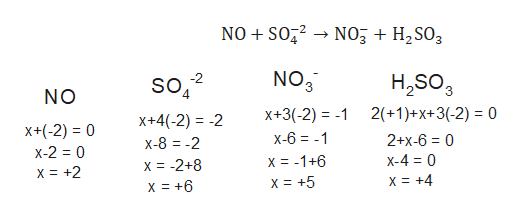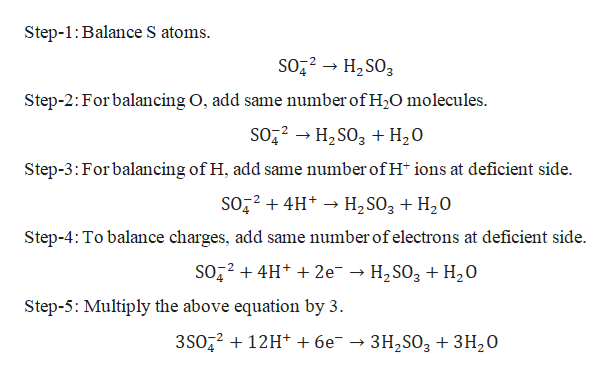# When the following equation is balanced properly under acidic conditions, what are the coefficients of the species shown?NO + SO42-NO3- + H2SO3What does Water appear as in the balanced equation as and with what  coefficient? How many electrons are transferred in this reaction?

Question

When the following equation is balanced properly under acidic conditions, what are the coefficients of the species shown?

NO + SO42-NO3- + H2SO3

What does Water appear as in the balanced equation as and with what  coefficient?

How many electrons are transferred in this reaction?

check_circleExpert Solution
Step 1

Balancing redox reaction by ion-electron method:

In the ion-electron method (also called the half-reaction method), the redox equation is separated into two half-equations - one for oxidation and one for reduction. Each of these half-reactions is balanced separately and then combined to give the balanced redox equation.

Steps for balancing a redox reaction:

1. Produce unbalanced equation for the reaction in ionic form.
2. Separate the equation into half-reactions.
3. Balance the atoms other than O and H in each half reaction individually.
4. For reactions occurring in acidic medium, add H2O to balance O atoms and H+ to balance H atoms.
5. Add electrons to one side of the half reaction to balance the charges. If need be, make the number of electrons equal in the two half reactions by multiplying one or both half reactions by appropriate number.
Step 2

Given reaction:

As N oxidation state changes from +2 to +5, it is undergoing oxidation by loosing electrons. And oxidation state of S changes from +6 to +4; it is undergoing reduction by gaining electrons.help_outlineImage TranscriptioncloseNO SO2NO H2SO3 NO3 H2SO 2(+1)+x+3(-2) 0 2 SO NO 4 x+3(-2) x-6 - X+4(-2) 2 x+(-2) 0 2+x-6 0 x-8 -2 x-2 0 x-4 0 x= -1+6 x= -2+8 x +2 x +4 x +5 x +6 fullscreen
Step 3

Balancing reduction h...help_outlineImage TranscriptioncloseStep-1: Balance S atoms SO22H2SO3 Step-2: For balancing O, add same number of H2O molecules SO22H2SO3 +H20 Step-3:For balancing of H, add same number ofH ions at deficient side. SO224H H2SO3 + H20 Step-4: To balance charges, add same number of electrons at deficient side SO224H 2e » H2SO3 + H20 Step-5: Multiply the above equation by 3 3SO2212H + 6e 3H2SO33H20 fullscreen

### Want to see the full answer?

See Solution

#### Want to see this answer and more?

Solutions are written by subject experts who are available 24/7. Questions are typically answered within 1 hour*

See Solution
*Response times may vary by subject and question
Tagged in

### Redox Reactions